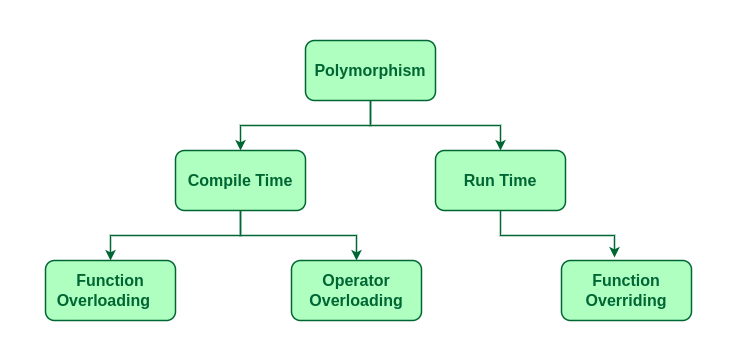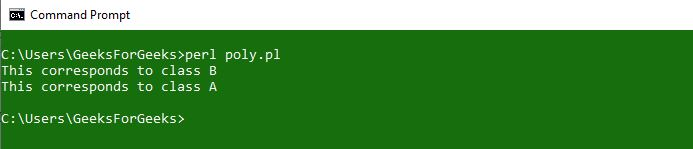GFG App
Open AppBrowser
Continue

# Perl | Polymorphism in OOPs

Polymorphism is the ability of any data to be processed in more than one form. The word itself indicates the meaning as poly means many and morphism means types. Polymorphism is one of the most important concepts of object-oriented programming languages. The most common use of polymorphism in object-oriented programming occurs when a parent class reference is used to refer to a child class object. Here we will see how to represent any function in many types and many forms. In a real-life example of polymorphism, a person at the same time can have different roles to play in life. Like a woman, at the same time is a mother, a wife, an employee and a daughter. So the same person has to have many features but has to implement each as per the situation and the condition. Polymorphism is considered as one of the important features of Object Oriented Programming.

Polymorphism is the key power of object-oriented programming. It is so important that languages that don’t support polymorphism cannot advertise themselves as Object-Oriented languages. Languages that possess classes but have no ability of polymorphism are called object-based languages. Thus it is very vital for an object-oriented programming language. It is the ability of an object or reference to take many forms in different instances. It implements the concept of function overloading, function overriding and virtual functions.Polymorphism

Polymorphism is a property through which any message can be sent to objects of multiple classes, and every object has the tendency to respond in an appropriate way depending on the class properties.

This means that polymorphism is the method in an object-oriented programming language that does different things depending on the class of the object which calls it. For example, \$square->area() will return the area of a square, but \$triangle->area() might return the area of a triangle. On the other hand, \$object->area() would have to calculate the area according to which class \$object was called. Polymorphism can be best explained with the help of the following example:

## Perl

 `use` `warnings;`   `# Creating class using package` `package` `A;`   `# Constructor creation` `sub` `new` `{` ` `  `    ``# shift will take package name 'vehicle' ` `    ``# and assign it to variable 'class'` `    ``my` `\$class` `= ``shift``;` `    ``my` `\$self` `= {` `                  ``'name'` `=> ``shift``,` `                  ``'roll_no'` `=> ``shift` `               ``};` `    `  `    ``sub` `poly_example` `    ``{` `      ``print``("This corresponds to class A\n");` `    ``}` `};`   `package` `B;`   `# The @ISA array contains a list ` `# of that class's parent classes, if any` `my` `@ISA` `= (A);`   `sub` `poly_example` `{` `  ``print``("This corresponds to class B\n");`   `}`   `package` `main;`   `B->poly_example();` `A->poly_example();`

Output:For the first output, For the first output, the method poly_example() defined in class B overrides the definition that was inherited from class A and vice-versa for the second output. This enables to add or extend the functionality of any pre-existing package without re-writing the entire definition of the whole class again and again. Thus making it easy for the programmer.

Polymorphism is the ability of objects to take on different forms or behave in different ways depending on the context in which they are used. In object-oriented programming (OOP), polymorphism is achieved through the use of inheritance, interfaces, and method overriding.

In Perl, polymorphism is primarily achieved through method overriding, which allows a derived class to provide its own implementation of a method defined in the base class. This allows objects of the derived class to be used interchangeably with objects of the base class, without the calling code having to know the specific type of object being used.

## Perl

 `#!/usr/bin/perl` `# your code here` `#!/usr/bin/perl`   `# Define a base class` `package` `Shape;` `sub` `new {` `    ``my` `\$class` `= ``shift``;` `    ``my` `\$self` `= {};` `    ``bless` `\$self``, ``\$class``;` `    ``return` `\$self``;` `}`   `# Define a method in the base class` `sub` `draw {` `    ``my` `(``\$self``) = ``@_``;` `    ``print` `"Drawing a shape\n"``;` `}`   `# Define a derived class` `package` `Circle;` `use` `base ``qw(Shape)``;`   `# Override the draw method in the derived class` `sub` `draw {` `    ``my` `(``\$self``) = ``@_``;` `    ``print` `"Drawing a circle\n"``;` `}`   `# Define another derived class` `package` `Square;` `use` `base ``qw(Shape)``;`   `# Override the draw method in the derived class` `sub` `draw {` `    ``my` `(``\$self``) = ``@_``;` `    ``print` `"Drawing a square\n"``;` `}`   `# Create objects of the derived classes` `my` `\$circle` `= Circle->new();` `my` `\$square` `= Square->new();`   `# Call the draw method on the objects` `\$circle``->draw();` `\$square``->draw();`

Output

```Drawing a circle
Drawing a square
```

In this example, we define a base class Shape with a draw method. We then define two derived classes Circle and Square, both of which inherit from the Shape class and override the draw method with their own implementation. Finally, we create objects of the Circle and Square classes and call the draw method on them, demonstrating polymorphism in action.

My Personal Notes arrow_drop_up